September Flash Sale - 5% OFF - Code: SEP5
Price Match Guarantee
Free Shipping on Orders Over \$50
No Minimum Order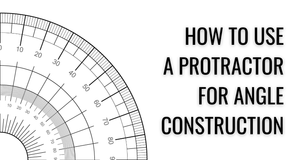# How to use a protractor for angle construction

The term angle construction refers to the process of drawing or constructing various angles with the help of instruments and mathematical steps. Constructing angles is considered to be one of the fundamental and basic concepts in geometry. Learning how to use a protractor for angle construction is thus an important skill that can be helpful in different ways.

In real-life applications, constructing angles is also vital in a number of projects and jobs. From installing a cabinet to building a high-rise, accurate angles are important to a wide variety of endeavors.

Below, we’ll be discussing the different kinds of angles and how to take accurate measurements using a digital or analog protractor. This can be helpful in different ways and can also possibly prevent you from making errors in your work.

# Angles: A general overview

Fundamentally, angles are a mathematical concept. They can seem intimidating, especially if you’re not all that well-versed in or comfortable with numbers. However, by being more familiar with the basics of angles and how they’re measured, they can be easier and less intimidating to work with.

## What are angles?

An angle is the space between two straight lines, also referred to as “rays,” that meet at a common endpoint. This endpoint is referred to as the “vertex” of the angle.

Angles are measured in degrees. To make understanding angles easier, it can be helpful to envision an imaginary circle with the vertex in the circle. Full circles measure 360 equal degrees. You can thus think of angles as sections of a full circle, or like slices of a pie.

## Importance of angles

By measuring angles accurately, you’ll be able to have a better understanding of how certain lines relate to each other. We learn a lot of mathematical concepts at school, some of which might not have much relevance to our present real-life activities. However, angles are quite literally everywhere, and they’re one mathematical concept that many of us deal with in everyday life.

Angle measurements have a lot of applications and are important to a lot of fields and professions. For example, athletes use them to determine how to make their shots, engineers use them when designing various structures, woodworkers use them when building furniture, and many more.

## Angle measurement tools

There are several ways to measure angles, and you can also use a variety of tools depending on what you’re currently working on. The following are some of the tools you can use for angle measurements:

• Analog protractors: You may remember using a small, transparent, and semi-circular piece of plastic to measure angles in geometry class. These protractors are quite basic tools but are still quite useful, especially for students. There are also more advanced versions of these protractors that are more suitable for professional use.
• Analog and digital angle finders: Typically, these angle finders have two arms that you can lay against the two rays of the angle you’re trying to measure. The digital versions have an LCD that will then display the exact measurement of the angle.
• Angle gauges: An angle gauge is a digital angle finder, but without the arms. It’s basically just a small box with a sensor that will determine the angle between two lines. Typically, it has magnetic sides that you’ll be able to easily affix on metal surfaces.
• Miter guide: A miter guide won’t give you exact measurements, but it can help ensure that miter cuts are easy and simple to make.
• Speed square: This is a triangular tool with one right angle. It’s usually used by carpenters and can also make measuring angles quick and easy to do while maintaining a good degree of accuracy.

# Types of angles

Certain angle measurements are known by certain names. Thus, they sometimes will just be referred to by their names, without the need to mention their exact measurements. The different types of angles are as follows:A right angle measures 90 degrees. Any two lines that flank a right angle are described as perpendicular. Right angles are also often illustrated with a small square at the vertex.

## Acute angle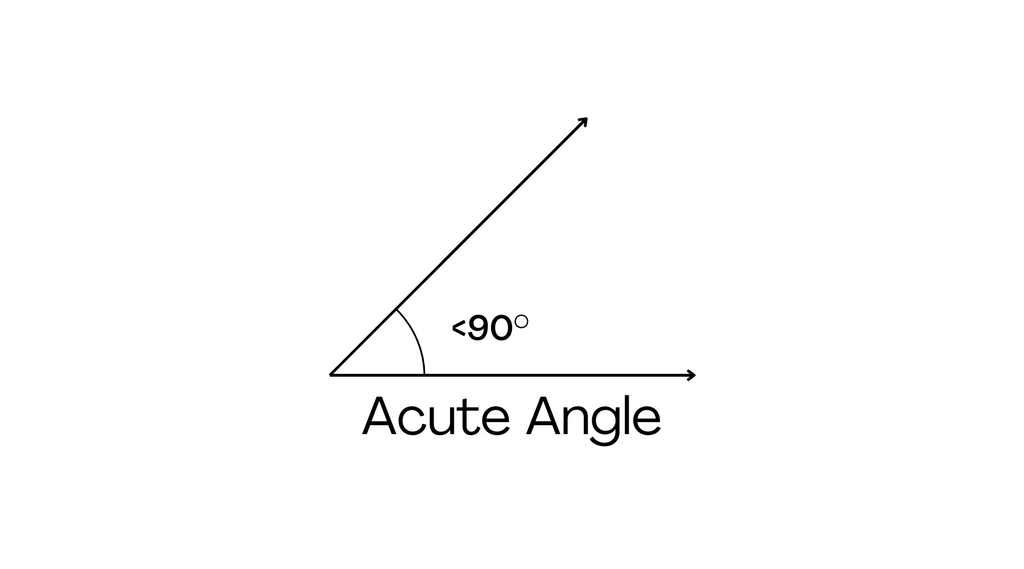Any angle that measures less than 90 degrees is described as an acute angle. Angles that measure 30, 45, 60, all the way up to 89 degrees are thus considered to be acute angles.

## Obtuse angle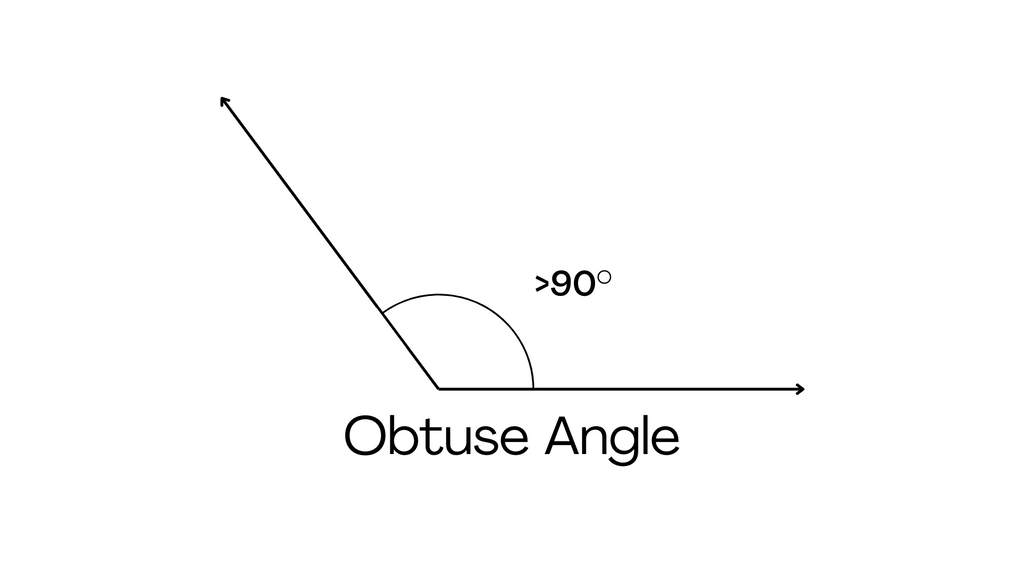While acute angles are angles that measure less than 90 degrees, obtuse angles are angles that measure over 90 degrees but measure less than 180 degrees.

## Straight angle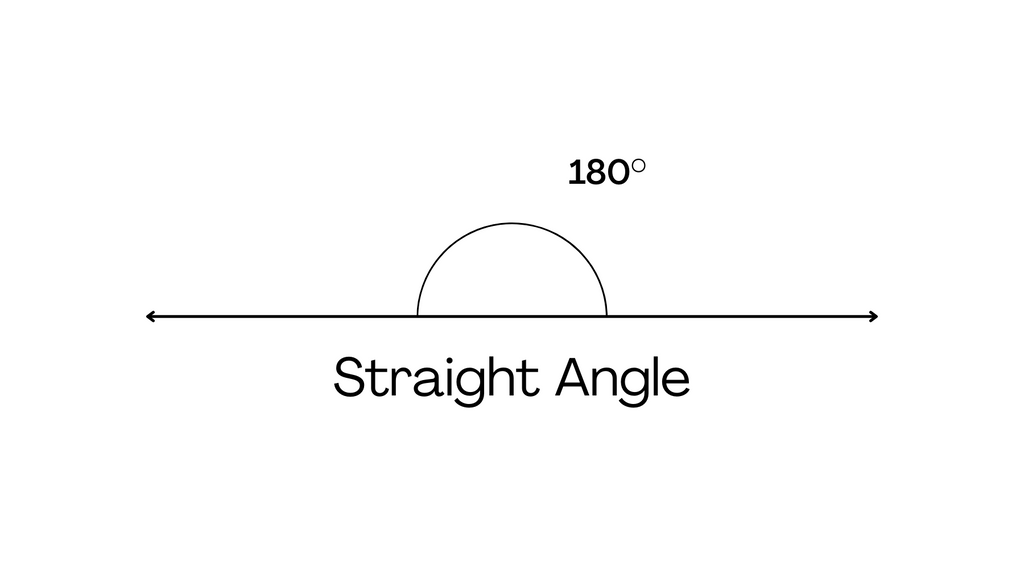A straight angle measures 180 degrees and is basically equivalent to two right angles. The two rays of these angles can point in two different directions but can also form a single straight line.

## Reflex angleMeanwhile, reflex angles are any angles that measure over 180 degrees but measure less than 360 degrees. These include angles that measure 200, 270, 300, all the way up to 359 degrees.

## Full angleA full angle is an angle that measures a full 360 degrees, basically forming a full circle. The two rays form a single straight line but are pointing in the same direction.

# How to use a protractor to construct angles

Because part of the purpose of a protractor is to determine specific measurements, it’s important for students to understand how to use one correctly. Here are the basic steps of using a protractor to measure and construct an angle.

1. To use a protractor for angle construction, first identify the vertex of the angle.
2. If you’re using a protractor with two arms, first align one arm with one of the rays of the angle.
3. Rotate the other arm until it lines up with the other ray forming the angle.
4. If you’re using an analog angle finder or protractor, read the markings on the tool to determine the measurement of the angle. If you’re using a digital tool, simply wait for the LCD to display the measurement.

This is only one approach to measuring angles. There are other methods available, and ultimately the method that you use will depend on the type of angle finder or protractor that you’re working with. Either way, practice will give you the skills you need to find angles quickly and easily.

Regardless of which method or measuring tool you use, however, certain things won’t change. You’ll have to work with the rays of the angle, and you’ll have to know how your angle measurement instrument works to make sure that you get the right results. Learning how to use a protractor to construct angles is easy enough, as long as you use the instrument correctly.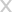## GET \$5 OFF

#### FOR YOUR FIRST ORDER INSTANTLY

I’m not interested in saving money on my order### Enjoy Your Coupon Code Now!

##### Shop with confidence, your satisfaction is guaranteed.
WELCOME5 SHOP NOW